14.15J | Spring 2018 | Undergraduate

# Networks

Lecture and Recitation Notes

## Recitation 9 Notes

### Topics

• Games with homogeneous externalities
• Games with local network effects

### Recall

Congestion games had simple sets of payoffs but complicated network structure. There are games that have complicated payoffs with simple network structure.

### Homogeneous Externalities

In classical economics, the demand curve is assumed decreasing and the supply curve increasing. The existence of network effects may create a weird shape of the demand curve.

• N = [0, 1] continuum of players
• ui(Si, S-i) = ui(Si, x) =
• vi ~ F([0, 1])
• p > 0
• Where x is how many buy, vi is its value, and p is price.

#### Example: Office suites, SNS, etc.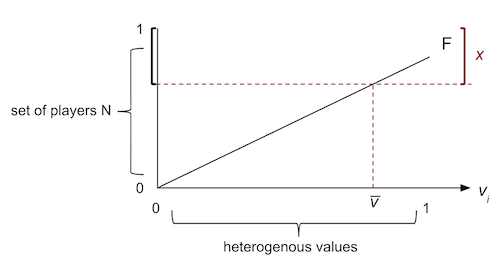• x = 1 — F() where  is the lowest value of agents who buy.

Claim: If agent with vi is better off buying, then any agent with vj is also better off buying.

Corollary: We may assume without loss of generality that x (those who buy) have the highest values in equilibrium/socially optimal outcome.

1. Socially optimal outcome
1. Social welfare = **∫1[v(1 — F()) — p] dF(v)
2. Maximizing this with respect to  yields the social best.
2. Nash equilibrum
1. Pooling equilibrum:
If no one has the good (x = 0) then no one has incentive to buy ( vi * 0 — p < 0). Therefore, x* = 0 is an equilibrium.
2. Separating equilibrium:
If someone buys (x > 0), then there exists the lowest type who buys. His incentive must balance v̅xp = 0.

Note that everyone’s strategy is summarized by x. So consider the aggregate best response function BR(x) = . With v̅x — p = 0 and x = 1 — F(), we find:

• BR(x) = 1 — F(p/x).
• Its fixed point x* is an equilibrium.

### Local Network Effects

Some games have both complicated payoff structure and complicated network structure.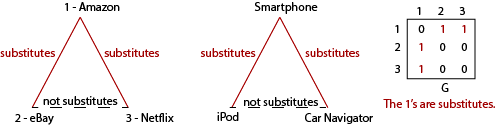• N = {1, 2, 3}.
• Si = ℝ+ = [0, ∞).
• ui(xi, x-i, δ, G) =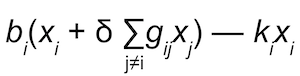i’s best response satisfies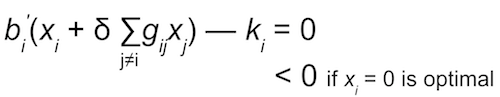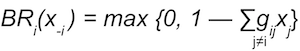• BR(x) = max { 𝟘, 𝟙 — δG𝕏}

## Course Info

Spring 2018
##### Learning Resource Types
Problem Sets with Solutions
Exams with Solutions
Lecture Notes